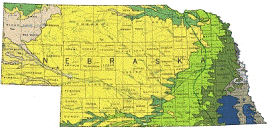## US Geological Survey## USGS Staff -- Published Research

#### Date of this Version

2004

Published in Advances in Water Resources 27 (2004) 547–562

#### Abstract

This study proposes the use of several problems of unstable steady state convection with variable fluid density in a porous layer of infinite horizontal extent as two-dimensional (2-D) test cases for density-dependent groundwater flow and solute transport simulators. Unlike existing density-dependent model benchmarks, these problems have well-defined stability criteria that are determined analytically. These analytical stability indicators can be compared with numerical model results to test the ability of a code to accurately simulate buoyancy driven flow and diffusion. The basic analytical solution is for a horizontally infinite fluid-filled porous layer in which fluid density decreases with depth. The proposed test problems include unstable convection in an infinite horizontal box, in a finite horizontal box, and in an infinite inclined box. A dimensionless Rayleigh number incorporating properties of the fluid and the porous media determines the stability of the layer in each case. Testing the ability of numerical codes to match both the critical Rayleigh number at which convection occurs and the wavelength of convection cells is an addition to the benchmark problems currently in use. The proposed test problems are modelled in 2-D using the SUTRA [SUTRA––A model for saturated–unsaturated variable-density ground-water flow with solute or energy transport. US Geological Survey Water-Resources Investigations Report, 02-4231, 2002. 250 p] density-dependent groundwater flow and solute transport code. For the case of an infinite horizontal box, SUTRA results show a distinct change from stable to unstable behaviour around the theoretical critical Rayleigh number of 4 π2 and the simulated wavelength of unstable convection agrees with that predicted by the analytical solution. The effects of finite layer aspect ratio and inclination on stability indicators are also tested and numerical results are in excellent agreement with theoretical stability criteria and with numerical results previously reported in traditional fluid mechanics literature.

COinS